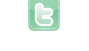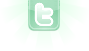## I need help writing some code....

Lets say there are 2 actors. Actor 1 and Actor 2. And lets give them a variable, Actor 1 is A and Actor 2 is B. So....there is a boolean, its called detectB.it is false. if (detectB= false, look forB in a certain radius . if a B is in a certain radius, detectB=true how would you make a code like that?
I'll write some of this as pusedo code, but you're wanting a basic if statement.
```private int ActorA = 0;
private int ActorB = 0
private boolean Detect_B = false;

// some method to store the if statement
public void DetectActorB()
{
If (!DetectB){